## 1. Street Sideline Intersection

 Two intersecting streets are shown on the right. The centerline direction for each is known as are their widths. Given the coordinates of a point on each centerline, what are the coordinates of the sideline intersection at point P?Since bearings are given, this is a bearing-bearing intersection. Because point P is not on the centerlines it should not be connected directly to the two given coordinate points. Instead, create two new points, G and H, on each sideline. These will serve as the baseline for the intersection triangle.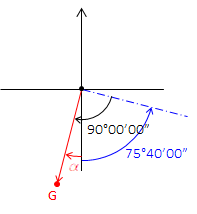To create point G, go perpendicular to the centerline at the given coordinate: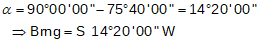Do the same to create point H:Inverse to determine the length and direction of the baseline GH:Sidelines have the same directions as their respective centerlines. Using those, compute the three angles:Then using the Law of Sines, determine the two missing sides: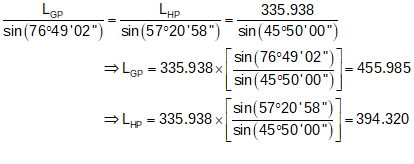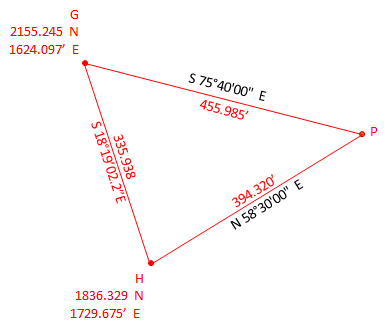Compute point P from point G:Math check - compute point P from point H: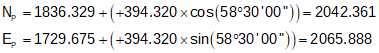check